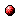# Max-plus-algebraic problems and the extended linear complementarity problem - Algorithmic aspects

Reference:
B. De Schutter, W.P.M.H. Heemels, and A. Bemporad, "Max-plus-algebraic problems and the extended linear complementarity problem - Algorithmic aspects," Proceedings of the 15th IFAC World Congress (b'02), Barcelona, Spain, pp. 151-156, July 2002.

Abstract:
Many fundamental problems in the max-plus-algebraic system theory for discrete event systems - among which the minimal state space realization problem - can be solved using an Extended Linear Complementarity Problem (ELCP). We present some new, more efficient methods to solve the ELCP. We show that an ELCP with a bounded feasible set can be recast as a standard Linear Complementarity Problem (LCP). Our proof results in three possible numerical solution methods for a given ELCP: regular ELCP algorithms, mixed integer linear programming algorithms, and regular LCP algorithms. We also apply these three methods to a basic max-plus-algebraic benchmark problem.Online version of the paperCorresponding technical report: pdf file (118 KB)
Note: More information on the pdf file format mentioned above can be found here.

Bibtex entry:

@inproceedings{DeSHee:01-15,
author={B. {D}e Schutter and W.P.M.H. Heemels and A. Bemporad},
title={Max-plus-algebraic problems and the extended linear complementarity problem -- {Algorithmic} aspects},
booktitle={Proceedings of the 15th IFAC World Congress (b'02)},